The role of oblivion, memory size and spatial separation in dynamic language games

Journal of Artificial Societies and Social Simulation vol. 5, no. 2
<https://www.jasss.org/5/2/1.html>

To cite articles published in the Journal of Artificial Societies and Social Simulation, please reference the above information and include paragraph numbers if necessary

Received: 3-Dec-2001      Accepted: 12-Mar-2002      Published: 31-Mar-2002

###Abstract

In this paper we present some multiagent simulations in which the individuals try to reach a uniform vocabulary to name spatial movements. Each agent has initially a random vocabulary that can be modified by means of interactions with the other agents. As the objective is to name movements, the topic of conversation is chosen by moving. Each agent can remember a finite number of words per movement, with certain strength. We show the importance of the forgetting process and memory size in these simulations, discuss the effect of the number of agents on the time to agree and present a few experiments where the evolution of vocabularies takes place in a divided range.

Keywords:
Agent-based Simulation; Communication; Language Games; Self-organisation

###1. Introduction

1.1
Agents in a multi-agent system need to share a common vocabulary in order to cooperate in any complete way. The lack of central control in such environments makes it difficult to reach an understanding between the agents. Sometimes, the system is open, in the sense that groups of agents can enter or leave the environment. This suggests that using an open, self-organising vocabulary could be an appropriate approach, providing the agents with mechanisms to handle this situation (Steels, 1998a).

1.2
Language games (Wittgenstein, 1974) and naming games simulations were introduced by Luc Steels (Steels 1996a) (Steels 1996b). In these systems, agents use extra-linguistic elements to point to the object they want to talk about, and they record information about all the words they have heard. These experiments take place in environments with (typically) about ten agents. Other approaches can be found in (Oliphant, 1996a) which uses genetic algorithms, (Baillie and Nehaniv, 2001) and (Billard and Dautenhahn, 1999) which use neural networks, (Oliphant and Batali, 1996b), (Kirby, 1998), (Hurford, 1999) and (Fyfe 1997). Interaction games are reviewed in (Nehaniv, 2000).

1.3
In a previous work with language games (de Lara and Alfonseca, 2000) we have experimented with moving and trusting strategies in much more populated agent communities than in Steel¥s naming games (400 to 1000 agents). Agents told one another both the word they were using and the strength of their belief that such a word is appropriate. This may not be very realistic, nor portable because agents are communicating their internal state. One can imagine a simulation in which agents are implemented using different techniques, and internal states can be meaningless for one another.

1.4
In this paper we have modified the agent model, so that communication of the internal state is no longer necessary. Agents are provided with a memory of finite size, and also with belief indicators. Unlike Steel's simulations, agents don't need to remember all the words they have heard. In fact, in our model we show that this is counterproductive to some degree. We also experiment with other simulation parameters, fixed in Steel's simulations, such as the memory size of each agent, the number of them, etc. We also show the importance of forgetting (weakening the confidence that each agent has in each word it knows) to reach an agreement quickly and propose several ways to accelerate this process. Finally we experiment with the moving strategies proposed in (de Lara and Alfonseca, 2000).

1.5
The last part of the paper presents some experiments on spatial separation. In these experiments we have separated two populations of agents, and let them communicate only through a narrow channel. A different approach, in which lexicon change is obtained through the addition of new agents to the simulation, can be found in (Steels and Kaplan 1998).

1.6
The rest of the paper is organised as follows: section 2 explains the basic organisation of our model; in section 3 we discuss the relevance of the forgetting process and show why it is necessary; section 4 deals with the importance of the memory size; section 5 shows the effect of the number of agents on the time to reach agreement; section 6 presents the results with different movement strategies; section 7 discusses some experiments in which agents are separated geographically, and finally section 8 explains the conclusions and suggests future work.

###2. Dynamic Language Games

2.1
A language game is an interaction between two agents involving a language (Steels 1998a). In this paper, we use the name dynamic language game (DLG) for a language game whose objective is to name movements, where the topic of conversation is chosen by moving. The chosen movement can be random or can follow a predefined strategy.

2.2
The objective of the DLG is to have the agents interact until they reach a common vocabulary to name each two-dimensional spatial movement (N, S, E, and W). The agents move in a grid of finite size, and more than one agent can be at the same location. Agents in the same location engage in a dialog about their chosen movement directions.

2.3
In all the experiments in this paper, agents will move in a 21x21 grid, usually connected at the sides (a plane torus). In most experiments, we will use 600 agents for the simulation.

2.4
The following is a pseudocode for the actions performed at each time step in the simulation.

```For-all agent (a): a chooses a movement direction
For-all agent (a): a moves according to the chosen direction
For-all agent (a):
For-all agent (b) such that b is located in the same position as a
a dialogs with b about a's chosen direction

```
Listing 1: pseudocode of the actions performed at each time step

2.5
This is, agents communicate in turns and communication is one-to-one. At each time step every agent involved in a DLG takes the roles of speaker and listener. The conversation topic is the speaker's last movement. In this way, agents can perceive and remember other agents' movements. The way in which agents choose the movement is random, but agents have several words associated with each movement. This is, each agent is provided with several memory locations to store words, together with their associated confidences. In our model, words are represented as integer numbers between 1 and 1000. The associated confidence is also a natural number, the higher this number is, the higher is the confidence in the word.

2.6
We let the agents learn several possibilities for each movement; i.e., the agents may associate more than one pair (word, confidence) to each movement. In this way, we allow synonymy and homonymy: An agent can associate the same word to several movements, and a movement can be associated to different words.

2.7
For example, Table 1 represents the words known by a certain agent, where the memory size is set to four, i.e. up to four possible words can be remembered for each movement. It can be noted that several positions are free pairs (0,0), that is, memory positions which are not occupied by any word. This may be the case if the agent has had few interactions with others (and has not filled all the positions) or if the words in some of the positions have been 'forgotten' (see section 3).

2.8
During the conversation between the agents, the listener talks about its last chosen movement. It selects one of the words associated to this movement with a probability proportional to its confidence with that word. In the situation shown in Table 2, and supposing that the agent moves to the North, word 128 would be chosen with a probability of 4/12, word 300 with a probability of 2/12 and word 987 with a probability of 6/12, as the sum of the indicators is 12.

Table 1: Example of a matrix of words and confidences for a particular agent
 Movement 1st word and confidence 2nd word and confidence 3rd word and confidence 4th word and confidence N (128, 4) (300, 2) (987, 6) (0, 0) S (2, 7) (30, 2) (504, 1) (300, 3) E (15, 2) (0, 0) (0, 0) (0, 0) W (657, 5) (226, 3) (0, 0) (0, 0)

2.9
An agent raises its confidence in a word (by adding one to the corresponding confidence) when it hears that word from another agent. If an agent hears a word for the first time, it may replace one of the words it knows (associated with the corresponding movement) with the new word. The agent can perform this operation if the word to be replaced has a confidence less or equal to one; or if the agent has a free memory position. The simulation starts with all the agents choosing a random word (one of 1000 different possibilities) for each movement, with an initial confidence of one.

###3. The Need for a Forgetting Process

3.1
The situation described in the previous section lacks negative feedback, i.e., the confidence values always grow. This can lead to deadlock situations, where agents are not able to reach an understanding. This situation can be originated by the initial agents' positions, as shown in figure 1: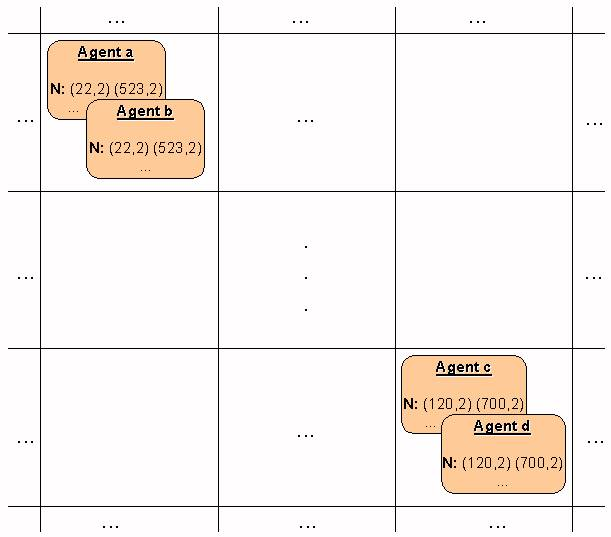Figure 1. Deadlock produced in a situation with no negative feedback (memory size=2)

3.2
In this picture, we can see that all the agents in the same spatial zone have filled every memory position with the same words. This originates a common vocabulary for the agents in the same zones, and as the agents have filled up all their memory locations with confidences greater than one, it will not be possible to agree on a global common vocabulary.

3.3
Thus, it seems necessary to add negative feedback to the model. We have experimented with three ways of doing this:

1. By augmenting the competition between the words used to name the same movement. That is, whenever an agent hears a word it has in its memory, it increases its confidence in it by one unit, but decreases its confidence in the others by the same amount (if that confidence was greater than one). This is a similar approach to (Steels and Kaplan, 1998), with a finite memory size. In 30 experiments with a memory size of 4 (i.e. 4 different words can be remembered for each movement), we have obtained an average time to agree (TTA) of 649 with a standard deviation of 184.7, a minimum of 406 and a maximum of 1152. In the following, we will call this approach "competition approach".
2. By means of forgetting. This means that at certain time steps, the confidence each agent has in all the words it knows is decremented by one. This makes it possible that common words in a zone become more popular, making the uncommon words disappear. In the following, we will call this approach "forgetting approach".

A problem with this approach is how to choose the appropriate forgetting rate. Figure 2 shows a comparison of different rates: 15, 25, 50, 75 with a memory size of 4. These are the intervals (number of time steps) at which the confidences are decreased. Each rate of forgetting was tested by 30 experiments. For rates less than 15 or greater than 75, the experiments never ended in 35000 time steps. The standard deviation is shown as error bars.

Looking at the graphic, it can be seen that the best fixed rate of forgetting is around 30. But, looking at the simulations, it can be observed that, for lower forgetting rates, convergence towards agreement accelerates in the final phase, whereas with higher forgetting rates the standard deviation decreases quickly at the beginning, but takes longer in the final time steps. This suggests it could be better to use a variable forgetting rate, in such a way that the agents forget slowly at the beginning, with the rate increasing as time goes by. For this purpose, we have used the hyperbolic-tangent time-dependent expression in figure 3 (where the x-axis is the time scale).Figure 2. TTA, depending on the forgetting rate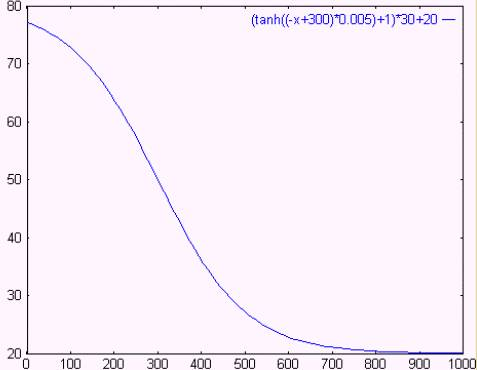Figure 3. Expression used for the forgetting rate

Using that expression, the agreement process is accelerated considerably. The following table shows a comparison with the fixed rate experiments.

Table 2: Time To Agree for fixed and variable forgetting rates
 Fixed at 75 Fixed at 50 Fixed at 25 Fixed at 15 Variable Average 3740.1 1858 1284.5 9324.47 873.74 Standard Deviation 6399.13 1921.51 595.11 1576.11 520.03 Minimum 552 450 660 6506 438 Maximum 29348 7180 4105 13498 2754

3. By combining the two previous approaches, in such a way that:

3.4
Comparing the three approaches, it is apparent that the competition-forgetting approach gives the better results, other parameters being equal. Results of a one-tailed t-test show that the competition-forgetting approach is better than the competition approach with an 85% confidence and better than the forgetting approach with a 99% confidence. A one-tailed Mann-Whitney U-Test shows that it is better than the competition approach with a 90% confidence and better than the forgetting approach with a 99.5% confidence.

###4. Memory size

4.1
In this section, we discuss the role of memory size (i.e. the number of words than can be remembered for each movement) in the agreement process for the three approaches of the previous section. At first sight, one could think that the larger the memory size, the faster the agreement process. This is not necessarily true, and the reason is the 'competition' between all the words used by the agent for a certain movement, which emerges because the word selected in a dialog depends on the confidence that the agent has in that word. Thus, the more words an agent can remember, the less frequently a word will be selected (the smaller its probability to be chosen).

4.2
On the other hand, if the agent can remember too few words for each movement, its confidence may not grow, because it will be unlikely that other agents it encounters use the same word, as each agent can remember very few words for each movement. Thus, there must be an equilibrium between 'inner' competition (competition between the words known by an agent) and 'outer' competition (competition between agents that try to impose their preferred words). These considerations resemble to some extent the ideas proposed in memetics (Dawkins 1976).

4.3
In the following subsections, we will show the TTA obtained in the simulations as a function of the memory size for each of the approaches presented in section 3. Each size has been tested by performing 30 experiments. It must be noted that, for the competition approach, we need at least a memory capacity of 2, because if the agents can remember only one word, deadlock situations can arise. The reason is that once the (only) word has a belief indicator greater than one, it cannot diminish, and no new words can be learned by the agents.

4.4
Table 3 and Figure 4 show the TTA, as a function of the memory size, for the competition approach. For smaller memory sizes, agreement was never reached in less than 35000 time steps. For a memory size of 3, there was an experiment that did not finish in less than 35000 time steps.

Table 3: Statistics for different memory sizes, competition approach.
 Memory Size 3 4 5 6 7 8 9 Average 3327.9 649 669.5 875.4 1098.5 1396.5 1864.5 Standard deviation 4747.9 184.7 126 110.2 182 280.8 419.8 Minimum 530 406 497 695 792 1001 1224 Maximum >35000 1152 1033 1211 1525 2010 3222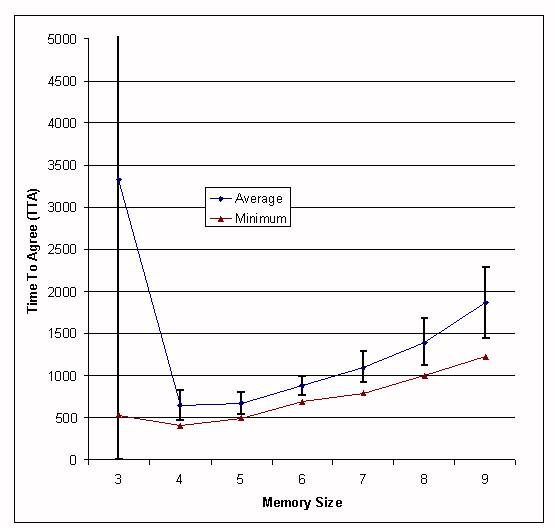Figure 4. TTA depending on the memory size, competition approach.

4.5
From these data, it seems that the better results are obtained with a memory size of 4, although results from a one-tailed t-test show that a memory size of 5 is worse with a 70% confidence. A Mann-Whitney U-Test shows that a memory 5 is worse with an 89% confidence.

4.6
Table 4 and Figure 5 show the same results for the forgetting approach. For larger and shorter memory sizes, agreement was never reached in less than 35000 time steps. It can be seen that the best results are also obtained with a memory size of four. A one-tailed t-test showed that a memory size of 4 is better than a memory size of 3 with a 99% confidence, and better than 5 with a 95% confidence. A Mann-Whitney U-Test showed that 4 is better than 3 with a 99.9% confidence and better than 5 with a 96% confidence.

Table 4: Statistics for different memory sizes, forgetting approach, with variable forgetting rate.
 Memory Size 2 3 4 5 Average 2223 1760.4 873.7 2026 Standard deviation 2409.9 1805.4 520 3653 Minimum 711 628 438 469 Maximum 9902 9001 2754 13971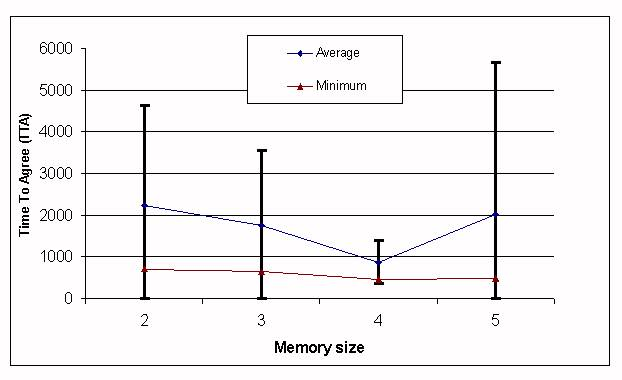Figure 5. TTA depending on the memory size, forgetting approach.

4.7
Table 5 and Figure 6 show the results of 30 experiments with different memory sizes, using the competition-forgetting approach. A one-tailed t-test shows that a memory size of 4 is better than a memory size of 3 with a 95% confidence, and better than a memory size of 5 with a 98.5% confidence. A Mann-Whitney U-Test showed that 4 is better than 3 with a 93.5% confidence and better than 5 with a 99.5% confidence.

Table 5: Statistics for different memory sizes, competition-forgetting approach.
 Memory Size 2 3 4 5 6 7 8 9 Average 787.3 705.6 597.6 673.5 949.2 1251.2 1767.5 2400 Standard deviation 167.3 264.9 163.2 135.6 186.5 256.8 440.3 591 Minimum 564 399 380 473 652 862 1013 1090 Maximum 1181 1390 1022 1210 1441 1933 3320 3673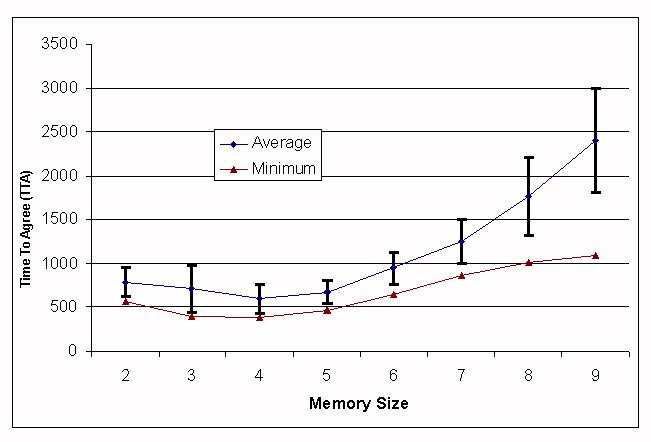Figure 6. TTA depending on the memory size, competition-forgetting approach.

4.8
Figure 7 shows a comparison of all the approaches. As it can be noted, in all of them the better results were obtained with a memory size of four. Therefore, for this size there seems to be an equilibrium between the 'inner' and the 'outer' competition. In other cases either the inner (five and above) or the outer (three and below) dominate. The competition-forgetting approach has given better results in terms of average TTA and standard deviation. Results of the statistical tests were shown at the end of section 3.

4.9
Also, it must be noted that the competition-forgetting approach number is better for small memory sizes (4 and below), whereas the competition approach is better for larger memory sizes (5 and above).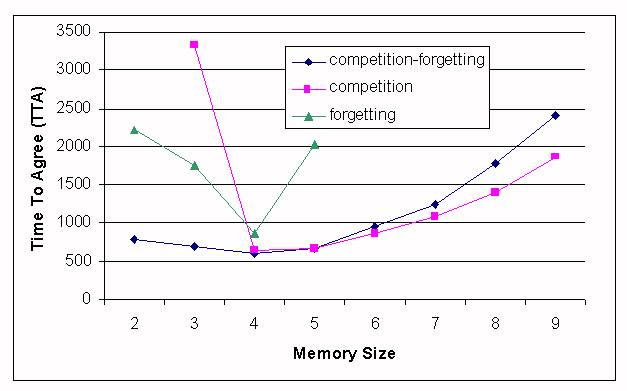Figure 7. Comparison of all the approaches

4.10
One may wonder whether these results depend on the number of different words initially in the population. Up to now, we had at most 600 different words, as initially each one of the 600 agents is given a word for each movement (randomly chosen between a set of 1000 possible words). We have also experimented by changing the size of this set in the range [10, 10000] obtaining always a result of 4 as the best memory size. It must be noted that in the case of 10 words, the TTA for some experiments increases noticeably.

4.11
One may also wonder whether it is a coincidence that the number of concepts to be learnt (4 words, one for each movement) is the same as the memory size (4 words can be remembered for each movement, 16 memory positions in total). To investigate this phenomenon, we extended the number of possible movements from 4 to 8, that is, we allowed the agents to move in diagonal, and to learn words for these movements. We used the competition-forgetting approach and obtained the results in figure 8: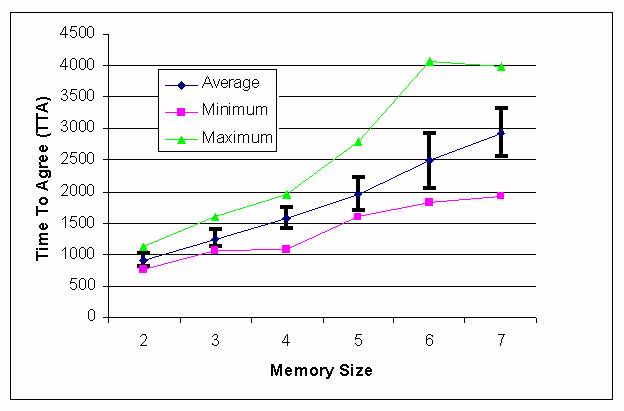Figure 8. TTA depending on the memory size, 8 concepts to be learned, competition-forgetting approach.

4.12
In this case, the best results are obtained with a memory size of 2 positions for each movement (16 memory positions in all, as in the previous case). The results with a memory size of 2 are better than those of a memory size of 3 with a 99.9% confidence using a one-tailed t-test and a one-tailed Mann-Whitney U-test.

4.13
One may also wonder whether the optimal memory size is also related to the agent density, that is, the number of agents divided by the size of the grid. We have performed experiments using the competition-forgetting approach with 300, 400, 600, 800, 1000, 1200 and 1400 agents in a grid of size 21x21. The size of the set from which the words are taken is kept equal to the number of agents in the simulation. The results of these simulations are shown in Table 6. It can be observed that the smaller the density, the smaller the optimal memory size.

Table 6: Statistics for different agent population densities, competition-forgetting approach.
 Population Density 0.68 0.907 1.361 1.814 2.268 2.721 3.175 Optimal memory Size 2 3 4 4 5 6 6 TTA 803.57 651.17 597.57 579.97 551.6 587.37 595.57 Standard deviation 238.72 163.1 160.42 157.86 189.96 156.93 189.61 Minimum 443 475 380 320 293 416 323 Maximum 1431 1244 1022 1052 1053 1143 1142

4.14
This table shows that an agent population density of 2.268 combined with a memory size of 5 gives the better results. This combination is better (using a one-tailed t-test) than a density of 1.814 with a 72% confidence and better than a density of 2.721 with a 79% confidence. A one-tailed Mann-Whitney U-test showed that it is better than a density of 1.814 with an 86% and better than a density of 2.721 with a 90.5% confidence. It must also be noted that for agent densities greater than 2.268 the TTA gets worse.

4.15
Next section explores more in detail the role the agent population density plays on the TTA.

###5. Number of agents

5.1
In this section, we will study the role that the number of agents in the simulation plays on the TTA. Basically, as the number of agents increases, the probability of occurrence of a DLG in a cell also increases. This is good, because it may accelerate the agreement process. If no DLG happens in a given time step, the step is wasted, because the system has not improved its coherence. Let N be the number of agents in the system and M the size of the territory in cells. Then, assuming randomly distributed agents, the probability of a particular cell (x,y) to be empty is:

Px,y (0) = ((M-1)/M)N

5.2
The probability of this cell to hold exactly one agent is:

Px,y(1) = (N/M)*((M-1)/M)N-1

5.3
Thus, the probability of a DLG to occur in a cell is:
Px,y(DLG)=1-(Px,y(0)+Px,y(1))
because at least two agents are needed for a DLG to take place. Figure 9 shows these probabilities with respect to the agent population density (number of agents divided by the number of positions in the grid).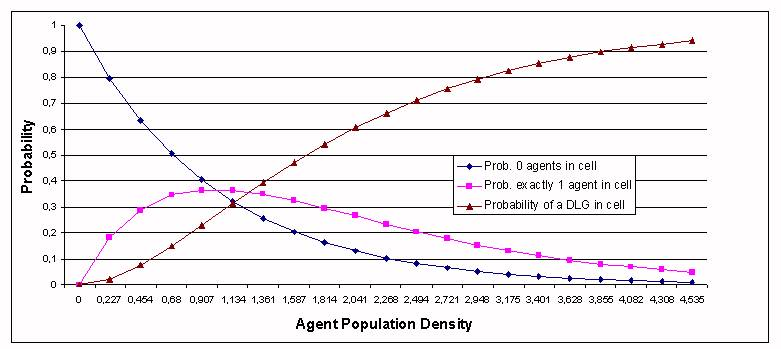Figure 9. Probability of a DLG in a particular cell as a function of the agent population density.

5.4
It must be noted that a DLG can involve more than two agents. The average of the number of agents involved in a DLG tends to grow with the number of agents. A greater number of agents in the system does not mean necessarily a greater TTA, as in each DLG each agent takes turns to "speak". If there are N agents in a cell, a particular agent will be involved in (N-1) interactions as a "speaker" and in (N-1) interactions as a "hearer", this gives 2*N*(N-1) interactions in the cell in a single time step. This increases the probability of reaching an agreement between the agents in the same cell.

5.5
Figure 10 shows the TTA obtained as a function of the agent population density. Thirty experiments were performed for each case. The experiments where performed with the competition-forgetting approach and a memory size of 4 (4 words can be remembered for each movement).Figure 10. TTA depending on the number of agents in the simulation, competition-forgetting approach.

5.6
From this figure, it can be observed that better results are obtained when the population density is greater than one, that is, when there are more agents than grid positions. But having in mind Table 6, it can be noted that for densities bigger than around 2.27 the TTA gets worse even with optimal memory sizes.

###6. Movement Strategies

6.1
In the preceding experiments, agents move randomly, therefore they have chosen their topic of conversation randomly. In this section we are going to analyse the effects of using other strategies to choose a word.

6.2
In a previous work (de Lara and Alfonseca 2000) we have shown that, in a set of experiments where the agents could remember only one word per movement, certain moving strategies reduce notably the agreement time. We presented two strategies:

• Best known word strategy (BNW): The agent's choice of direction depends directly on its confidence in the associated word, i.e. the higher the confidence in a word, the higher the probability of that movement to be chosen.
• Teach me strategy (TM): The agent chooses the movement associated with the word in which it has the least confidence. After moving, each agent requests the associated word from other agents sharing the same position.

6.3
For the competition approach, the BNW strategy was worse than the random version, whereas TM gave better results. In 30 experiments we obtained an average TTA of 596.2, with a standard deviation of 79.8, a minimum of 483 and a maximum of 861. A one-tailed t-test showed that the TM strategy is better than random movements with a 92% confidence. A Mann-Whitney U-test showed that the TM strategy is better with a 73% confidence.

6.4
For the forgetting approach, the BNW strategy is also bad, whereas TM results are comparable to the best case so far obtained for this model. In 30 experiments with this strategy, agreement was reached in an average of 948.37 steps, with a standard deviation of 595.8. The maximum was 3383, and the minimum 508.

6.5
For the competition-forgetting approach, both BNW and TM gave bad results. For example, for the TM strategy, in 30 experiments we obtained an average TTA of 638.4 and a standard deviation of 83, with a minimum of 506 and a maximum of 822. A one-tailed t-test showed that this strategy is worse than random movements with an 86% confidence, whereas a Mann-Whitney U-test showed that the TM strategy is worse with a 98% confidence.

6.6
Thus, one can infer that, except for the competition approach, the use of strategies to select the conversation topic does not reduce the TTA. That is, it is better for the agents to choose the conversation topic randomly.

###7. Spatial Separation

7.1
In this section, we examine several situations where the population is separated by one or more obstacles in the agents' world. The world is no longer a plane torus, its boundaries are not connected. The objective of these experiments is to see whether the agents develop a single vocabulary or a number of different ones. These experiments have been carried out using the competition-forgetting approach.

7.2
In the first set of experiments, we have set an obstacle in the middle of the territory. This obstacle has an opening that permits the agents to change sides. We have measured the averages and the standard deviation of the populations at both sides of the obstacle, in a rectangle two units below the obstacle's opening. Figure 11 shows the configuration for these experiments.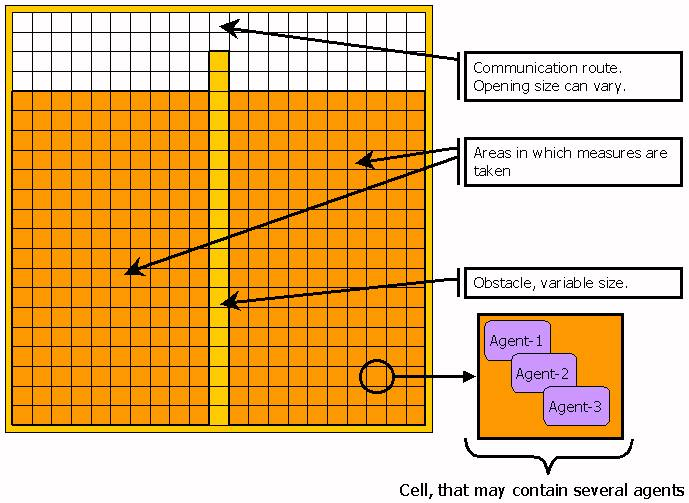Figure 11. Configuration of the experiments with spatial separation

7.3
In experiments with an opening of width two at one end, the populations at both sides develop different vocabularies initially. However, due to the agents that pass from one side to the other, little by little the vocabulary of both populations approach each other, until both sides reach a common vocabulary.

7.4
In these simulations, the time to reach an agreement is very high. For example, using the competition-forgetting approach, in 30 experiments we obtained an average of 13385.5 and a standard deviation of 3277.4 (a minimum of 7538 and a maximum of 23909). Similar results where obtained with the competition approach. With the forgetting approach, in all experiments we obtained a TTA greater than 35000 time steps.

7.5
Figure 12 shows the evolution of the word associated with "West" for the two populations in one of these experiments (using the competition-forgetting approach).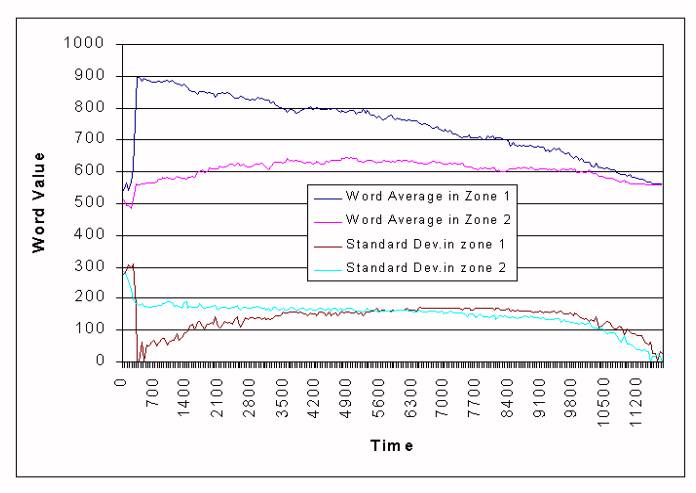Figure 12. Evolution of the word for "West" in a population divided in two zones.

7.6
In our models words are represented as natural numbers. Thus, the Y-axis in this graphic represents word values. The graphic shows the average of the word values for each population. We can distinguish two areas in the graphic:

• The first one, from step 0 to about 300 is a short, transitory state of the system in which several words compete in each zone to become dominant. At the end of this period, word 897 becomes dominant in the first region, while word 559 is dominant in the second zone, with a higher standard deviation in the second case, because other words also compete for dominance.
• The second period goes from step 300 up to step 11700. This is a period in which both words 559 and 897 try to become dominant in both zones. At the end of this period, word 559 is dominant in both zones.

7.7
If the opening is smaller (1 unit) the agreement time increases: in some experiments, agreement is never reached. These experiments can be seen as intermediate between the totally open case (no obstacles) and the totally closed (two different experiments, with agreement times for each area as in the normal case).

7.8
In a second set of experiments, we set a population divided in two totally separated sections and leave it evolve until agreement is reached on both sides (usually on different languages). Then a path is opened through the obstacle, allowing both populations to mix. For a period of time after the path is opened, the languages in both sides remain unchanged, despite agents changing sides. Later the dominant words of both zones begin to compete. After some time, a single hybrid language is created and becomes dominant, some words taken from the language at one side of the obstacle, some words from the other side.

7.9
The results of this last set of simulations are somehow similar to those described in Steels and Kaplan (1998). In their experiments, once a lexicon has been formed, new agents are incorporated to the simulation. They describe a period of stability (despite these new agents), followed by a period of instability, in which the new agents propagate the variations of their lexicon, and by another period of stability.

###8. Conclusions and Future Work

8.1
In this paper, we have presented a more realistic model than (de Lara and Alfonseca, 2000). We have also shown the importance of adding negative feedback to the agents, without which the agents may not be able to agree, because deadlock situations can arise. We have proposed three ways of modelling the negative feedback, the first by augmenting the competition between synonymous words, the second by a process similar to forgetting, and the third a mix of the previous two, where forgetting is not applied to the dominant word. If the agents choose the topic of conversation randomly, this last approach is better than the first one (with a confidence of 85% and 90% using parametric and non-parametric tests), the second approach is the worst. In this case, better results are obtained if the forgetting rate follows a hyperbolic-tangent expression.

8.2
Memory size plays a very important role in the agreement process. We have shown that, for the situation proposed, it has an optimal value of four for all the approaches. If it is larger, inner competition arises between the words inside an agent; if it is too small, outer competition (competition between the agents trying to impose their words) dominates. Both competition phenomena can play a detrimental role in the agreement process. The number of concepts to be learnt and the agent population density have also an influence on the TTA.

8.3
The number of agents is important. If there are too few, the probability of a DLG in a cell is low, and the number of interactions at each time step is also low. This makes the standard deviation for the TTA be high. As the number of agents is increased, DLGs become more frequent, together with the number of agents involved in each game. In a game, every agent takes the role of "speaker", therefore the number of interactions at each time step raises. But if the agent density is too high (above 2.27), the TTA gets worse.

8.4
With respect to the movement strategies, we have found 'teach-me' as presented in (de Lara and Alfonseca, 2000) the only one appropriate for the competition approach. This means that, in general, it is better for the agents to choose the conversation topic randomly.

8.5
The spatial separation experiments have shown that different vocabularies are not obtained if the populations are not completely separated, although they can initially develop a different vocabulary, that finally converges. The convergence process can be significantly slower than in the previous cases.

8.6
The model presented in this paper is limited by the number of concepts than can be learnt, as concepts are associated with movements. In the future, we will detach the referents of the words from the directions in which the agents move. We plan to integrate our communication model in a simulation of artificial ant colonies (Alfonseca and de Lara, 2002). In these simulations, there are several anthills whose ants communicate food positions directly, rather than by dropping pheromones. We have used these simulations to investigate several phenomena in knowledge propagation. Ants belonging to different nests don't communicate, thus compete for food. If the communication model proposed in this paper were integrated with those simulations, we could study the possible emergence of a shared, inter-nest vocabulary. For such integration, more complex semantic domains should be considered (not only spatial movements). It would also be useful to provide agents with rudimentary syntax rules for communication.

###Acknowledgements

The authors would like to thank three anonymous referees for their useful comments. This paper has been sponsored by the Spanish Interdepartmental Commission of Science and Technology (CICYT), project numbers TEL1999-0181, and TIC 2001-0685-C02-01.

###References

ALFONSECA, M., DE LARA, J., 2002. "Simulating evolutionary agent communities with OOCSMP". Proc. 17th ACM Symposium on Applied Computing (SAC'2002), track "AI and Computational Logic". Madrid, March 2002.

BAILLIE, J, NEHANIV, C., "Deixis and the Development of Naming in Asynchronously Interacting Connectionist Agents", Proceedings of the First International Workshop on Epigenetic Robotics, Lund University. Cognitive Studies, vol. 85, pp. 123-129, 2001.

BILLARD, A., DAUTENHAHN, K. "Experiments in Learning by Imitation - Grounding and Use of Communication in Robotic Agents", Adaptive Behavior. 1999, 7(3), 411-434.

DAWKINS, R. 1976. "The Selfish Gene". New York, Oxford University Press.

de LARA, J., ALFONSECA, M. 2000. "Some strategies for the simulation of vocabulary agreement in multi-agent communities". Journal of Artificial Societies and Social Simulation vol. 3, no. 4, <https://www.jasss.org/3/4/2.html>.

FYFE, C., Livingstone, D. 1997. "Developing a Community Language". ECAL¥97, Brighton, UK.

HURFORD, J. 1999. "Language Learning from Fragmentary Input". In Proceedings of the AISB'99 Symposium on Imitation in Animals and Artifacts. Society for the Study of Artificial Intelligence and Simulation of behavior. pp.121-129.

KIRBY, S. 1998. "Language evolution without natural selection: From vocabulary to syntax in a population of learners"'. Edinburgh Occasional Paper in Linguistics EOPL-98-1.

NEHANIV, C.L. 2000. "The Making of Meaning in Societies: Semiotic & Information-Theoretic Background to the Evolution of Communication". In Proceedings of the AISB Symposium: Starting from society - the application of social analogies to computational systems. pp. 73-84.

OLIPHANT, M. 1996. "The Dilemma of Saussurean Communication". BioSystems, 37(1-2), pp. 31-38.

OLIPHANT, M., BATALI, J. 1996. "Learning and the Emergence of Coordinated Communication". Center for Research on Language Newsletter 11(1).

STEELS, L. 1996a. "Self-organizing vocabularies". In C. Langton, editor. Proceedings of Alife V, Nara, Japan. 1996.

STEELS, L. 1996b. "A self-organizing spatial vocabulary". Artificial Life Journal. 2(3) 1996.

STEELS, L. 1998a. "The Origins of Ontologies and Communication Conventions in Multi-Agents Systems". Autonomous Agents and Multi-Agents Systems, 1, 169-194. STEELS, L, KAPLAN, F. 1998b. "Spontaneous Lexicon Change". In COLING-ACL98, pages 1243--1249. ACL, Montreal, 1998.

WITTGENSTEIN, L. 1974. "Philosophical Investigations". Basil Blackwell, Oxford.